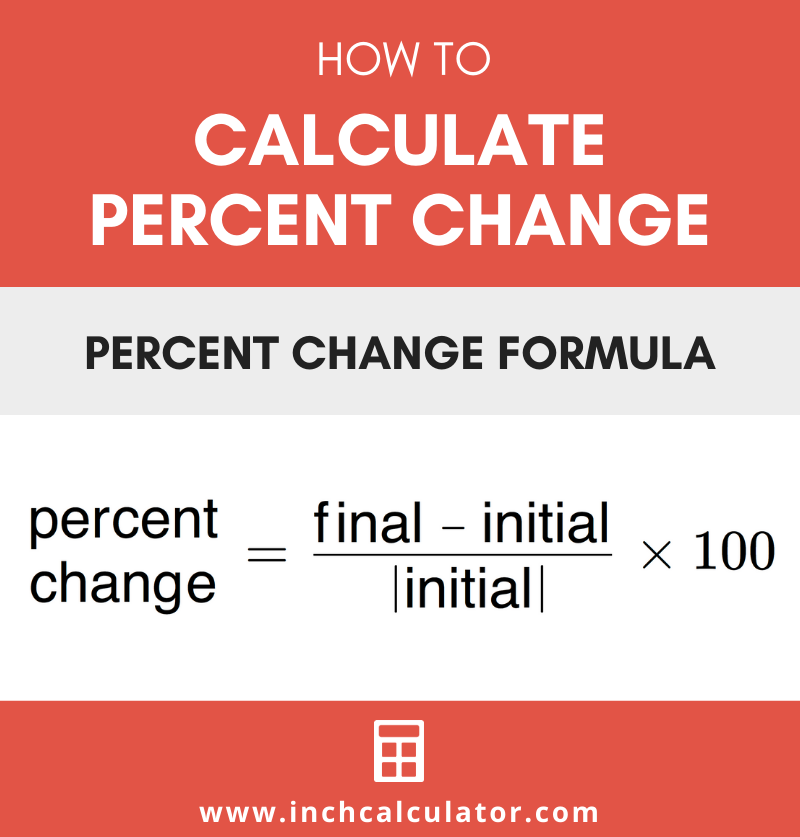# Percent Change Calculator

Calculate the percent of change between two numbers. A positive result indicates a percent change increase, while a negative result is a decrease.

## Percent Change:

300% increase

### Steps to Solve Percent of Change

p=(y2 - y1) / |y1|×100
Substitute values in the equation
p=
(60 - 15) / |15|×100
Simplify
p=
45 / 15×100
p=3×100
p=300%
Learn how we calculated this below

## How to Calculate Percent Change

Percent change is a measure of the degree of change between two numbers. The percent of change is a comparison of the old and new values.

For example, the percent of change from 2 to 4 is a 100% increase.

### Percent Change Formula

The percentage of change can be found using a simple formula.

p = y2 – y1 / |y1| × 100

Thus, percent change p is equal to the final value y2 minus the initial value y1, divided by the absolute value of the initial value y1, times 100.

So, to calculate percent change, substitute the initial and final values in the formula, then solve.Example: For an initial value of 50 and final value of 100, find the percentage of change

p = (y2 – y1) ÷ |y1| × 100
p = (100 – 50) ÷ |50| × 100
p = 50 ÷ 50 × 100
p = 1 × 100
p = 100%

Here’s a tip: if you know the value is increasing try our percent increase calculator.

For negative numbers, it is important to note that the absolute value of y1 should be used as the denominator. The absolute value is the value of the number without a positive or negative sign.

For example, the absolute value of both 50 and -50 is 50.

Example: For an initial value of -25 and final value of -250, find the percentage of change

p = (y2 – y1) ÷ |y1| × 100
p = (-250 – -25) ÷ |-25| × 100
p = -225 ÷ 25 × 100
p = -9 × 100
p = -900%

If the final value is decreasing, our percent decrease calculator works best.

## How to Calculate Percent Change Over Time

The percentage of change can also be measured over time. To calculate change over time, first, calculate the percent change using the formula p = y2 – y1 / |y1| × 100.

Then, divide the result by the time interval to calculate the percent change over the time period.

## Percent Change vs. Percent Difference

You might be wondering how percent change is different from percent difference?

Percent of change is an expression of the amount of change from an old value to a new value. On the other hand, percent difference is an expression of the difference between two values rather than the change.

For example, percent change might be used to express the change in value of an item as it increases from \$10 to \$20. Percent difference might be used to express the difference between one item costing \$10 and another costing \$20.

If you’re trying to find the difference between two values, try our percent difference calculator.

You can easily use our calculator above to solve percent change or use the formula to find it manually. And you might also find our percentage calculator useful for calculating percentages.